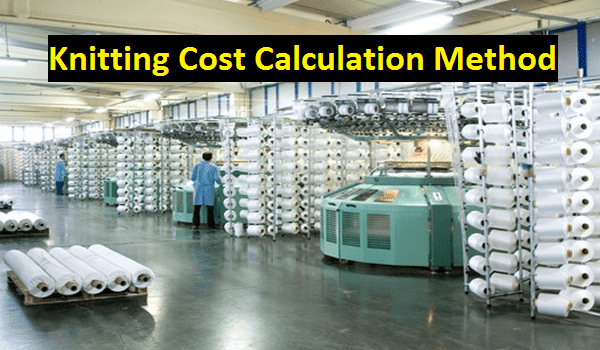# Apparel Costing

Solution of textile and apparel calculation

## How to Calculate Knitting Cost in Textile Industry?

Knit Fabric Cost and Knitting Cost Calculation in Textile:
Knitting cost calculation is too much important to achieve greater output in textile business. It is not an easy task that we have normally seemed. This article will help you to learn the exact calculating method for knit fabric cost and knitting rate calculation.Fig: Knitting cost calculation method in textile
Knitting Cost Calculation Method in Textile Sector:
Find out the knitting cost & rate and knitted fabric cost & price from the below chart.

 Parameters Amount or Quantity Total machine 2 Shift per day 3 Production capacity per shift 70kg per machine Yarn used 1000kgs Yarn price Rs. 200 per kg Knitting wastage 2% Operator wages Rs. 200 per shift Helper wages (In production) Rs. 100 per shift Inspection wages Rs. 200 per shift 1 helper 2 machines 1 operator 1  machine Inspection worker 1 person Power Rs. 4000 per month Rent Rs. 8000 per month Transport Rs. 6000 per month Salary Rs. 25000 per month Other expenses Rs. 24000 per month Profit 10%

Solution:
To calculate the knitting cost and rate we have to follow the below knitting cost calculation formulas:

1. Fabric Manufacturing and Selling Cost Calculation:

Knit fabric cost/kg,

Direct material (D.M) + Direct labor (D.L) + Direct expenses (D.E) + Overhead (OH)
= ……………………………........…………………………………….....……...................…      ….(1)
Total production

2. Knit Fabric Manufacturing Cost calculation:

Knitting cost/kg,

Direct labor (D.L) + Direct expenses (D.E) + Overhead (OH)
= ………………………...…………………………………………...……        ………….. (2)
Total production

Total Production Calculation:

Total production,
= Yarn used –wastage%
= 1000-2%
= 1000-20
= 980kg

Total Production Time Calculation:

Total production time = Total production/production capacity per shift …………………… (3)

Now,
Production capacity/shift,
= No. of machines × capacity
= 2×70
= 140kg

Now, from equation (3), we get,
Total production time,
= 980/140
= 7 shift

Direct Material (D.M) Cost calculation:

Yarn cost,
= 1000 × Rs. 200
= Rs. 200000

Direct Labor (D.L) Cost calculation:

Operator wage = No. of operator × No. of shifts × Rs. 200 = 2×7×200 = Rs. 2800
Helper wage = No. of helper × No. of shifts × Rs. 100 = 1×7×100 = Rs. 700
Inspection wage = No. of inspector × No. of shifts × Rs. 100 = 1×7×200 = Rs. 1400

So,
Direct labor (D.B) cost,
= Rs. 2800+ Rs. 700+ Rs. 1400
= Rs. 4900

Direct Expense (D.E) Cost Calculation:
Direct expense = 0

In case of power,
= (Cost × 7 shifts)/ (30days× 3 shifts in a day) = {(4000×7)/90} = Rs. 311

In case of rent,
= (Cost × 7 shifts)/ (30days× 3 shifts in a day) = {(8000×7)/90} = Rs. 622

In case of transport,
= (Cost × 7 shifts)/ (30days× 3 shifts in a day) = {(6000×7)/90} = Rs. 467

In case of salary,
= (Cost × 7 shifts)/ (30days× 3 shifts in a day) = {(25000×7)/90} = Rs. 1944

In case of others expense,
= (Cost × 7 shifts)/ (30days× 3 shifts in a day) = {(24000×7)/90} = Rs. 1867

So,
= Rs. 311+ Rs. 622+ Rs. 467+ Rs. 1944+ Rs. 1867
= Rs. 5211

Now, from equation (1), we get,
Knit fabric cost/kg,
= {(200000+4900+0+5211/980)} = Rs. 214.40

After adding 10% profit, knit fabric cost stands at,
= {214.40/ (1-10%)}
= Rs. 238.20

Again,
From equation (2), we get,
Knitting rate or knitting cost per kg,
= {(4900+0+5211)/980}
= Rs. 10.30

After adding 10% profit, knitting rate stands at,
= {10.30/ (1-10%)}